### Home > CAAC > Chapter 13 > Lesson 13.IF1-S > Problem6-11

6-11.
1. Solve each of the following equations for x. Homework Help ✎

1.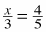2.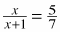3.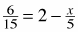4.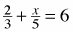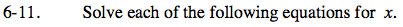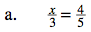$\textit{x}=\frac{12}{5}$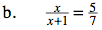$\textit{x}=\frac{5}{2}$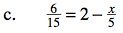Multiply both sides of the equation by 15.

6 = 30 − 3x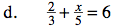See part (c).